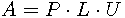Next: , Previous: Polynomials in Bernstein form, Up: Numerical algorithms

#### 5.2.3 Matrix routines

Geometrical library implements algorithms for matrix manipulation. The main feature is a linear equations system solver, using PLU factorization. Linear systems are not used very often in the project and appears only in computations with conic sections.

PLU factorization is the factorization of rectangular matrix A to a permutation matrix P, a lower triangular matrix L with ones on diagonal and an upper trapezoidal matrix U such that, U has the same size as A. P and L are square matrices with as many rows as A. The implementation of this algorithm by Gaussian elimination can be found in the file geomlib/matrix.c.

There are also routines solving the matrix kernel, rank, inversion or multiplication of two matrices.